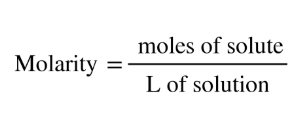# Problem: A chemist adds 470.0 mL of a 8.8 x 10 -5 mM silver(II) oxide (AgO) solution to a reaction flask. Calculate the mass in micrograms of silver(II) oxide the chemist has added to the flask. Round your answer to 2 significant digits.

95% (6 ratings)
###### FREE Expert Solution

Recall that molarity is equal toConvert the provided V to L from mL and mM to M. Multiply them to get the moles of AgO and then use the molar mass of AgO to find the mass in g. Lastly convert this g to microgram

95% (6 ratings)###### Problem Details

A chemist adds 470.0 mL of a 8.8 x 10 -5 mM silver(II) oxide (AgO) solution to a reaction flask. Calculate the mass in micrograms of silver(II) oxide the chemist has added to the flask. Round your answer to 2 significant digits.

What scientific concept do you need to know in order to solve this problem?

Our tutors have indicated that to solve this problem you will need to apply the Molarity concept. You can view video lessons to learn Molarity. Or if you need more Molarity practice, you can also practice Molarity practice problems.

What is the difficulty of this problem?

Our tutors rated the difficulty ofA chemist adds 470.0 mL of a 8.8 x 10 -5 mM silver(II) oxide...as medium difficulty.

How long does this problem take to solve?

Our expert Chemistry tutor, Sabrina took 6 minutes and 21 seconds to solve this problem. You can follow their steps in the video explanation above.

What professor is this problem relevant for?

Based on our data, we think this problem is relevant for Professor Veige's class at UF.